# Group Short Run Xbar-s Charts

Group short run Xbar-s charts enable you to spot changes in the process average and standard deviation across multiple characteristics in a limited production run. Review the following example—an excerpt from Innovative Control Charting1—to get a sense of how a group short run Xbar-chart works.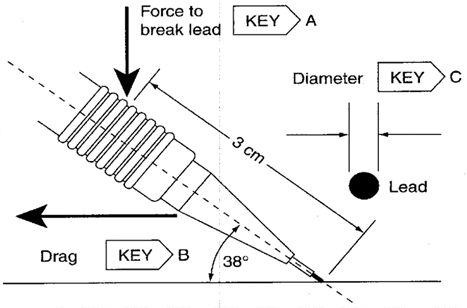Figure 1. Mechanical pencil with three key characteristics.

## Case Description

A company manufactures mechanical pencil lead. There are three key characteristics (see Table 30.5).

1. Break force—The amount of pressure it takes to break the lead (extended 1.5 mm) at a 38° angle with the force applied 3 cm from the lead rip
2. Drag—A proprietary measure of how smoothly the lead releases onto a given paper
3. Diameter—The diameter of the lead

Table 1. Upper and lower specification limits for three mechanical pencil lead key characteristics.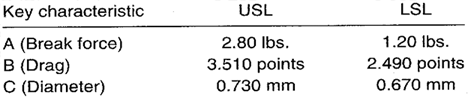The manager wishes to monitor the stability of all three key characteristics on the same chart.

## Sampling Strategy

Because production volume is very high and three different characteristics are to be monitored, a group short run Xbar-s chart is selected. Ten leads are tested every 30 minutes.

## Target Values

Preliminary tests on all three key characteristics were conducted. The purpose of the tests was to establish target values for the group short run charts to be used. The target values are found in Table 2.

Table 2. Target X and target s values for the three mechanical pencil lead key characteristics.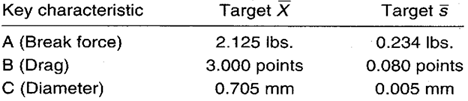## Data Collection Sheet

Table 3. Data collection sheet for the group short run Xbar-s chart pencil lead example. MAX and MIN plot points are shown in bold.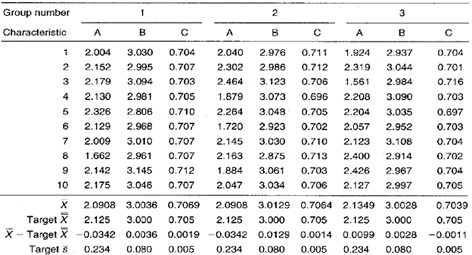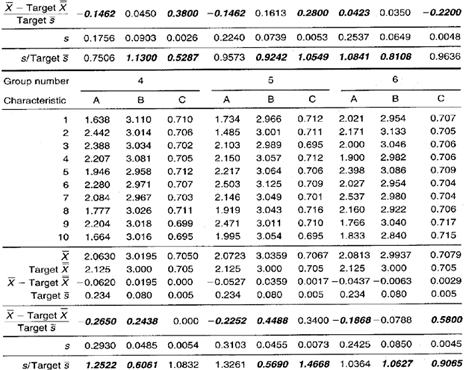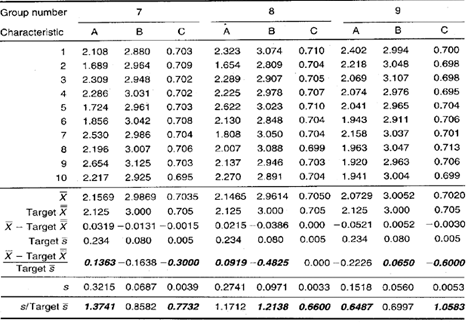## Group Short Run Xbar-s Chart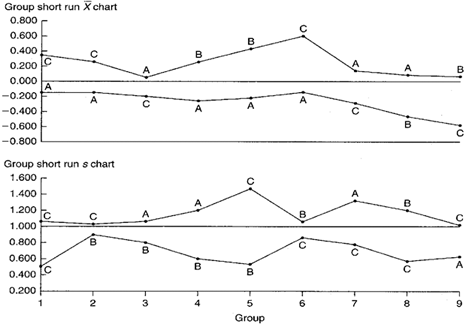Figure 2. Group short run Xbar-s chart for the pencil lead example. Three key characteristics are being monitored on the same chart.

## Chart Interpretation

Group short run s chart: All three characteristics—break force (A), drag (B), and lead diameter (C)—appear to randomly fluctuate in the MAX and MIN positions. This indicates that the initial target s values were good estimators for all of the characteristics.

Group short run Xbar chart: It appears that all three key characteristics are randomly fluctuating in the MAX and MIN positions. This means that the initial target values were good estimators of the actual means for each of the three characteristics.

## Recommendations

Group short run s chart: Continue using the initial target s values for all three characteristics. The charts may look good, but only the capability studies will determine if the characteristics are meeting engineering requirements.

Group short run Xbar chart: Continue using the initial target X values. No recalculation is necessary. The process averages appear stable and predictable. Continue to collect data. If the process remains stable, reduce sampling frequency.

## Estimating the Process Average

Estimates of the process average should be calculated separately for each characteristic on each part on the group short run charts. The estimate of the process average for break force can be found in Calculation 1.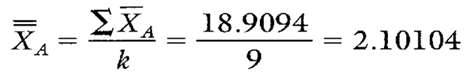Calculation 1. Estimate of the process average for characteristic A, break force.

## Estimating Sigma

Estimates of sigma are also calculated separately for each characteristic on each part on the group short run charts. Continuing with characteristic A, see Calculations 2 and 3.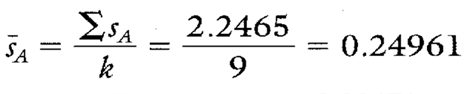Calculation 2. s calculation for characteristic A, break force.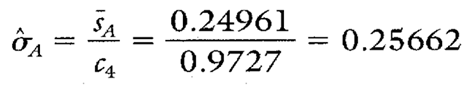Calculation 3. Estimate of the process standard deviation for characteristic A, break force.
Note: To ensure reliable estimates of both the process average and process standard deviation, k needs to be at least 20. In this example, k is only nine. Therefore, the estimates here and in Table 4 are shown only for illustration purposes.

## Calculating Process Capability and Performance Ratios

Calculations 4, 5, and 6 show the capability calculations for break force, characteristic A.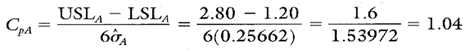Calculation 4. Cp calculation for characteristic A, break force.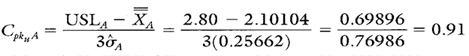Calculation 5. Cpk upper for characteristic A, break force.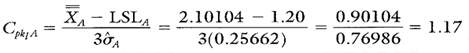Calculation 6. Cpk lower calculation for characteristic A, break force.

## Group Short Run Xbar-s Chart Advantages

• Graphically illustrates the variation of multiple product or process characteristics relative to each other.
• Characteristics from different parts with different means, different standard deviations, and different units of measure can all be analyzed on the same chart.
• Separates variation due to changes in the average from variation due to changes in the standard deviation.
• Separates variation due to the process from variation that is product specific.

## Group Short Run Xbar-s Chart Disadvantages

• No visibility of characteristics that fall between the MAX and MIN plot points
• Cannot detect certain nonrandom conditions because the group charts described here have no control limits
• Lots of calculations

Additional statistics and process capability and performance calculations for key characteristics B and C are shown in Table 4.

Table 4. Additional statistics and process capability and performance calculations for the drag and diameter key characteristics.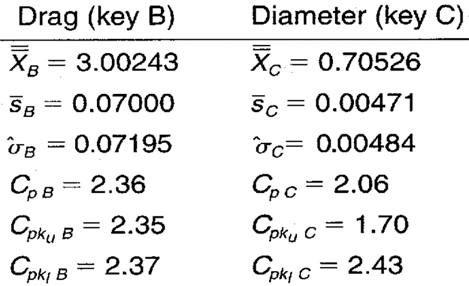When you use SPC software from InfinityQS, consuming the information provided by group short run Xbar-charts becomes faster and easier than ever. See how this type of analysis is surfaced in InfinityQS solutions.

FOOTNOTE:
1 Wise, Stephen A. and Douglas C. Fair. Innovative Control Charting: Practical SPC Solutions for Today’s Manufacturing Environment. Milwaukee, WI: ASQ Quality Press.

### Take the first step from quality to excellence

Take the Next Steps